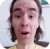FreeKB - Addition Subtraction Multiplication and Division in BASH
Addition Subtraction Multiplication and Division in BASH``````#!/bin/bash

x=10
y=2

echo \$((x + y))``````

Subtraction.

``````#!/bin/bash

x=10
y=2

echo \$((x - y))``````

Division.

``````#!/bin/bash

x=10
y=2

echo \$((x / y))``````

Multiplication.

``````#!/bin/bash

x=10
y=2

echo \$((x * y))``````

Let's say file1.txt contains a number of lines where each line is an integer.

``````0
10
0
20``````

The following command will add up all of the values in the file. In this example, the sum will be 30.

``````~]# awk '{s+=\$1}END{print s}' file1.txt
30``````

We will never share your name or email with anyone. Enter your email if you would like to be notified when we respond to your comment.

Please enterin the box below so that we can be sure you are a human.